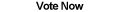Home Articles Components Code Snippets Links

C# Code Snippets# Point in Triangle

Kerry Millen

The traditional method is to use barycenter coordinates (Example 1). By using parameterized triangle line segments, an interpolated point may be found (Example 2).

Platform: .NET Framework 1.1+

``` (Example 1)
private Point getPointBaryCenter(Point p1, Point p2, Point p3)
{
return new Point((p1.X + p2.X + p3.X) / 3,
(p1.Y + p2.Y + p3.Y) / 3);
}

(Example 2)
private Point getInterpPt(Point p1, Point p2, Point p3)
{
return new Point((p1.X >> 1) + ((p2.X + p3.X) >> 2),
(p1.Y >> 1) + ((p2.Y + p3.Y) >> 2));
}

An explanation of how this works follows.
(p1, p2, and p3 are points of a triangle)

Get an interpolated pt some distance (t) between p1 and p2 and call it p12.
t is any number > 0 and < 1
p12 = p1 + t * (p2 - p1)

Get an interpolated pt some distance (t) between p1 and p3 and call it p13.
p13 = p1 + t * (p3 - p1)

Get an interpolated pt some distance (t) between p12 and p13 and call it pT.

(if 0 < t < 1, this point must be inside the triangle)
pT = p12 + t * (p13 - p12)

Substitute 0.5 for t and reduce (t can be any value).

0.5 * p1 + 0.25 * (p2 + p3)
(p1 >> 1) + ((p2 + p3) >> 2)

My evaluation of this method found it to be between 2.5% and 4.4% faster than the barycenter method.```

Back to C# Code Snippet List